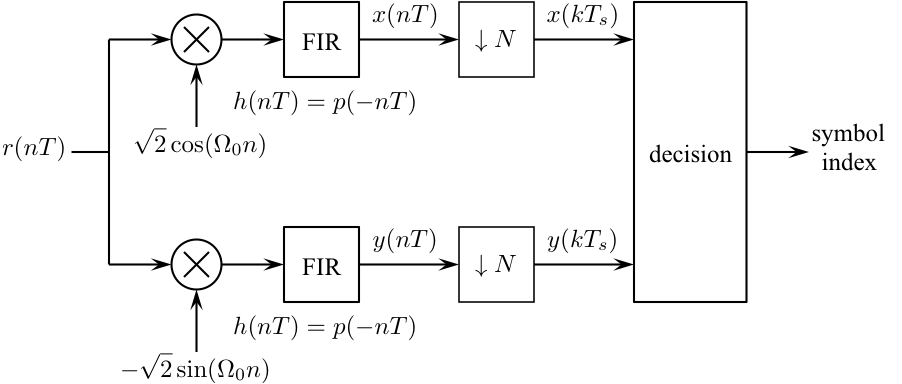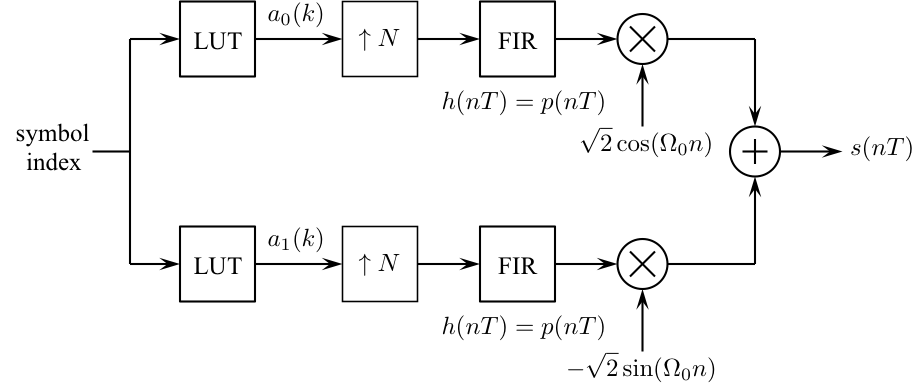# Digital Communications: A Discrete-Time Approachby Michael Rice# Quaternary Phase Shift Keying (QPSK)

## Introduction

Quaternary Phase Shift Keying, QPSK, is one of the most popular digital carrier modulations in use today. The QPSK signal set consists of four waveforms that differ in phase: they are each 90-degrees apart. For this reason, the QPSK constellation consists of four points equally spaced on a circle. In this exercise, you will design QPSK detector to process the data contained in the file qpskdata.mat

## Textbook References

M-ary QAM: Section 5.3 (pp. 238 - 260), discrete-time realizations: Section 5.3.2 (pp. 256 - 260), partial response pulse shapes: Section A.2 (pp. 682 - 687).

## Specificationsnormalized sample rate: 8 samples/symbol normalized carrier frequency: 0.25 cycles/sample carrier phase: 0 degrees average energy: 9 pulse shape: SRRC (50% excess bandwidth, span = 12 symbols) symbol clock offset: 0 input file qpskdata.mat input message length: 56 symbols (112 bits or 16 ASCII characters)

## Preliminary Design

### Design the Detector

Design the detector, shown below, using blocks from the Simulink, DSP System, and Communications System Toolboxes.### Test the Detector Design

You should test the detector you designed by constructing a modulator to produce a test signal. The following procedure steps you through this design process:
1. Design the modulator shown below to meet the above specifications except make the input the four symbol sequence 0 2 1 3.2. Connect the output of your modulator to the input of your detector.

3. Connect the output of your detector to a To Workspace block (be sure to open the Properties Dialog Window and set the Save format to matrix) and a Scope block.

4. Set the simulation parameters as follows:
 Simulation Time
 Start Time: 0.0 Stop Time: (12+4)*8-1
Solver Options
 Type: Fixed-step Solver: discrete (no continuous states) Fixed step size: auto
 Periodic sample time constraint: Unconstrained Tasking mode for periodic sample times: SingleTasking
Note: the stop time is computed as follows: 12 = 2*(span/2) [the delay of the pulse shaping filter plus the delay of the matched filter]; 4 = the number of data symbols; 8 = the number of samples/symbol. We subtract because the start time is t = 0.0.

5. Run the simulation and plot the demodulator input and the matched filter output on the same set of axes. The simulation produces 16 downsampled matched filter outputs -- the last 4 correspond to the sequence (0 2 1 3). Check the values in the workspace to see if they agree with input sequence (0 2 1 3).

6. Adjust the offset of the Downsample block to obtain the proper values.

## Exercise

1. Replace the modulator blocks with the From File block and set the Filename to qpskdata.mat and the sample time to 1.

2. Set the simulation parameters as follows:
 Simulation Time
 Start Time: 0.0 Stop Time: (12+56)*8-1
Solver Options
 Type: Fixed-step Solver: discrete (no continuous states) Fixed step size: auto